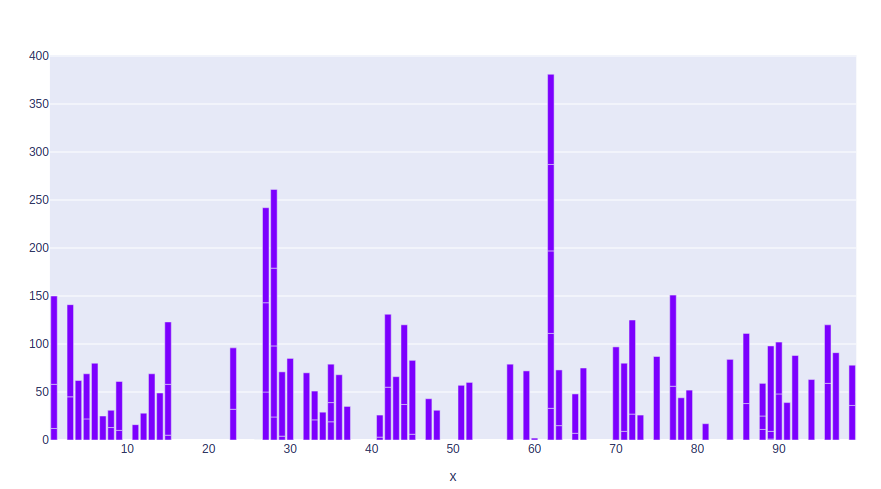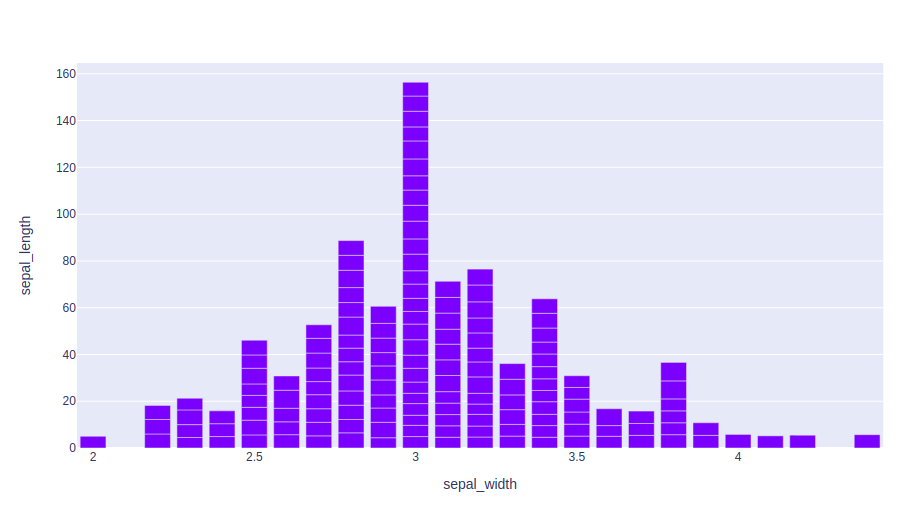# Bar chart using Plotly in Python

Plotly is a Python library which is used to design graphs, especially interactive graphs. It can plot various graphs and charts like histogram, barplot, boxplot, spreadplot, and many more. It is mainly used in data analysis as well as financial analysis. plotly is an interactive visualization library.

## Bar Chart in Plotly

Bar chart with plotly express is much convenient and   high-level frontier to plotly, which helps to operate diversification of data and which helps to produce easy-to-style figures. With px. bar, each row in the DataFrame is represented as a rectangular mark, it can be horizontally or vertically. Alls helps to show comparisons among discrete categories. One of the axis in the chart shows the specific categories being compared, and the other axis represents a measured value. It can be created using the bar() method of plotly.express.

Syntax: plotly.express.bar(data_frame=None, x=None, y=None, color=None, facet_row=None, facet_col=None, facet_col_wrap=0, hover_name=None, hover_data=None, custom_data=None, text=None, error_x=None, error_x_minus=None, error_y=None, error_y_minus=None, animation_frame=None, animation_group=None, category_orders={}, labels={}, color_discrete_sequence=None, color_discrete_map={}, color_continuous_scale=None, range_color=None, color_continuous_midpoint=None, opacity=None, orientation=None, barmode=’relative’, log_x=False, log_y=False, range_x=None, range_y=None, title=None, template=None, width=None, height=None)

Parameters:
data_frame: DataFrame or array-like or dict needs to be passed for column names.
x: Either a name of a column in data_frame, or a pandas Series or array_like object. Values from this column or array_like are used to position marks along the x-axis in cartesian coordinates.
y: Either a name of a column in data_frame, or a pandas Series or array_like object. Values from this column or array_like are used to position marks along the y axis in cartesian coordinates.
color: Either a name of a column in data_frame, or a pandas Series or array_like object. Values from this column or array_like are used to assign color to marks.
hover_data: Either a list of names of columns in data_frame, or pandas Series, or array_like objects or a dict with column names as keys, with values True

Example 1:

## Python3

 `import` `plotly.express as px ` `import` `numpy ` ` `  `# creating random data through randomint  ` `# function of numpy.random  ` `np.random.seed(``42``)  ` `   `  `random_x``=` `np.random.randint(``1``,``101``,``100``)  ` `random_y``=` `np.random.randint(``1``,``101``,``100``) ` ` `  `fig ``=` `px.bar(random_x, random_y) ` `fig.show()`

Output:Example 2: Using the iris dataset

## Python3

 `import` `plotly.express as px ` ` `  `# Loading the iris dataset ` `df ``=` `px.data.iris() ` ` `  `fig ``=` `px.bar(df, x``=``"sepal_width"``, y``=``"sepal_length"``) ` `fig.show()`

Output:My Personal Notes arrow_drop_upIf you like GeeksforGeeks and would like to contribute, you can also write an article using contribute.geeksforgeeks.org or mail your article to contribute@geeksforgeeks.org. See your article appearing on the GeeksforGeeks main page and help other Geeks.

Please Improve this article if you find anything incorrect by clicking on the "Improve Article" button below.

Article Tags :

Be the First to upvote.

Please write to us at contribute@geeksforgeeks.org to report any issue with the above content.